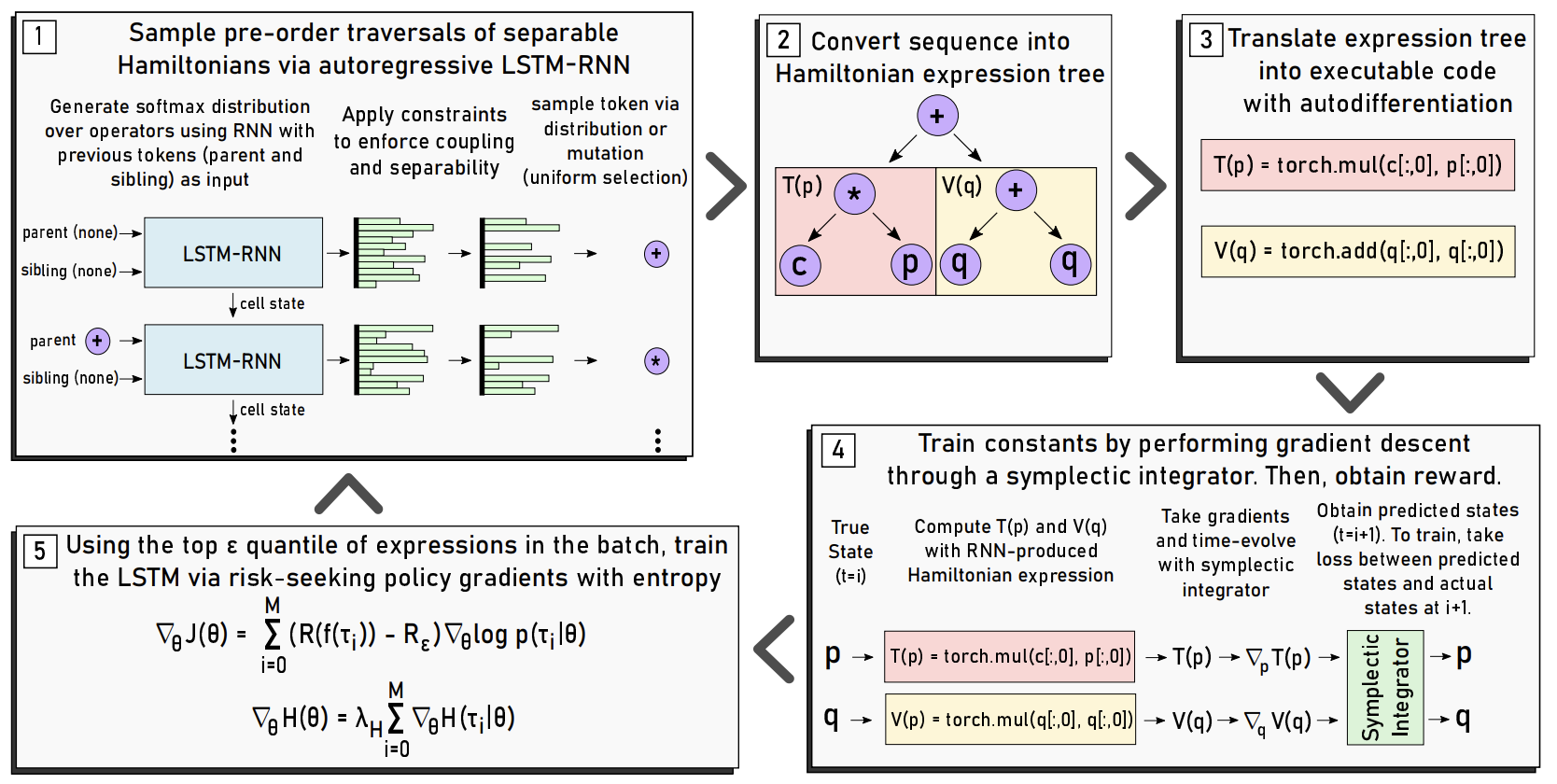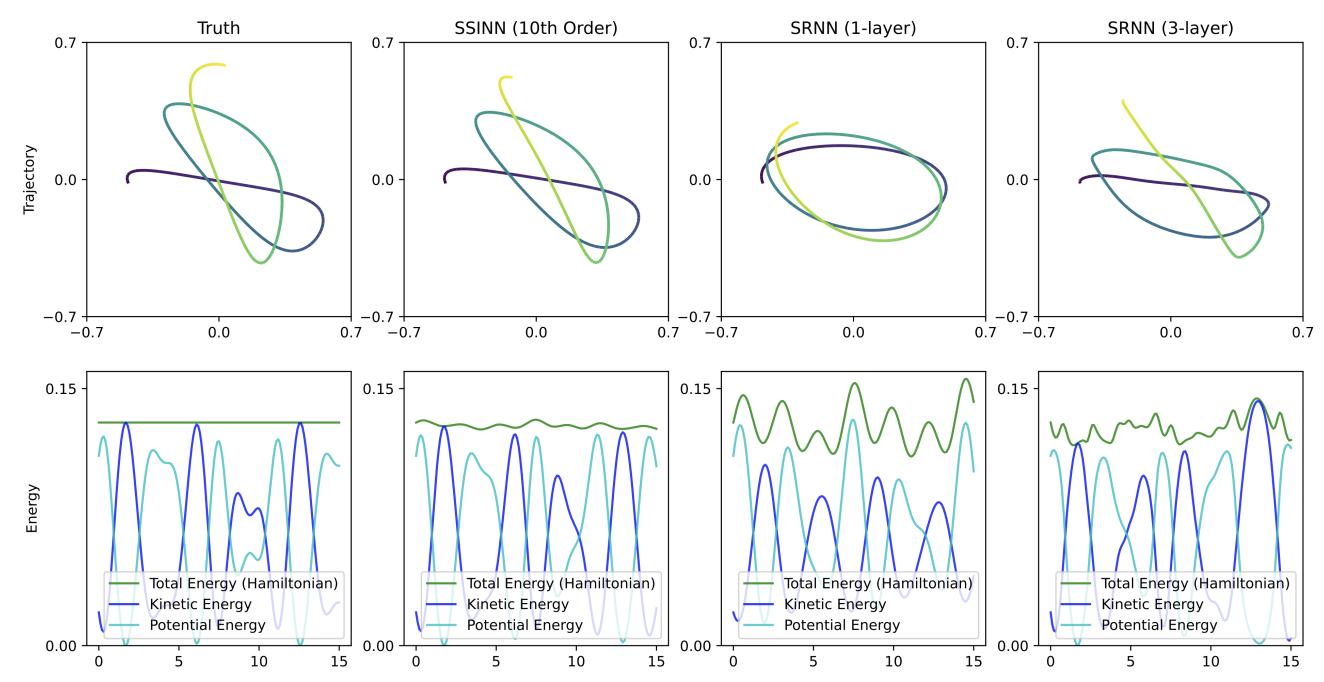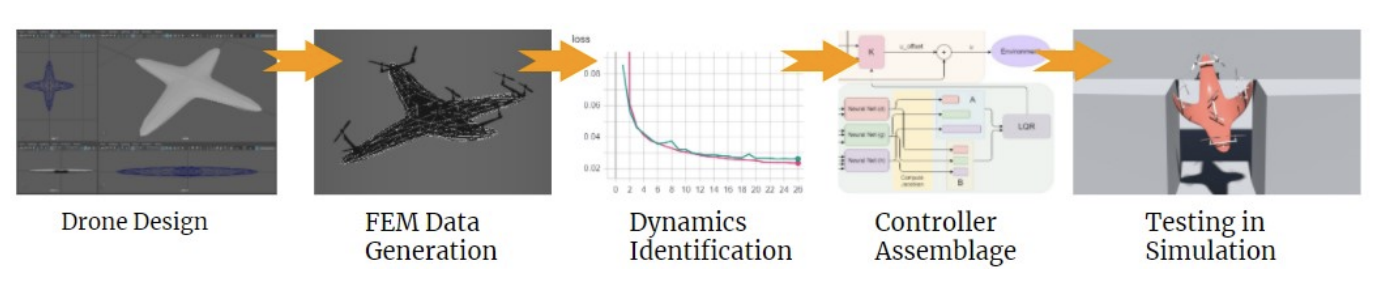I enjoy lots of flavors of research. My favorite problems combine novel mathematical thinking with challenging computational implementations.

I will do my best to provide high-level summaries of my work here–they probably won’t make sense without domain knowledge. I think there’s no substitute for reading the abstract or the paper itself (most of these are freely available).

## Machine Learning (Computational Physics)#

DiPietro, D. M., & Zhu, B. (2022). Symplectically Integrated Symbolic Regression of Hamiltonian Dynamical Systems. arXiv:2209.01521. Paper.• Fundamental Question: How can we learn symbolic governing equations from observational data of physical dynamical systems?
• We present a novel machine learning framework for answering this question. It works in the following way:
1. We use some specially constructed symplectic neural networks to deduce algebraic properties of the governing equations, such as additive separability.
2. Next, an LSTM-RNN autoregressively generates a sequence of operators/system variables that corresponds to the pre-order traversal of an expression tree for some symbolic equation. Specifically, the LSTM-RNN is structured to generate separable physics equations called Hamiltonians.
3. We transpile the expression trees into Pytorch code so that we can optimize their constants with auto-differentiation.
4. We use the expression tree to time-evolve the physical system by symplectically integrating it. The error that we get in doing so is our loss function.
5. Due to the transpiling step, we lose end-to-end differentiability. So, we train the LSTM-RNN with a reinforcement learning approach called risk-seeking policy gradients.
6. We go back to step 2 and repeat until we’re satisfied with the generated equation.
• Code: https://github.com/dandip/SISR
• My Contribution: This was my senior thesis–I’m responsible for the implementation, nearly all of the ideation, and the paper. My advisor suggested step 1, which greatly improved performance. The variable-length batching code of the LSTM-RNN, as well as the transpiler, presented fun technical challenges.

DiPietro, D. M., Xiong, S., & Zhu, B. (2020). Sparse Symplectically Integrated Neural Networks. Advances in Neural Information Processing Systems. Paper.• Fundamental Question: How can we learn symbolic governing equations from observational data of physical dynamical systems?
• We present a novel machine learning framework for answering this question. It works in the following way:
1. Pick some finite function space, i.e. 6th order polynomials, perhaps with some trigonometric terms.
2. Implement a neural network that encodes this function space, with the coefficients of each term being trainable parameters.
3. To obtain loss, symplectically integrate the neural network (making this a form of neural differential equation) and take the L1-error between its predicted future state of the physical system and the physical systems actual future state. Train the network until it converges (you have your equation) or fails to (pick a different function space).
• Code: https://github.com/dandip/ssinn
• My Contribution: My co-authors helped with the numerical integration code. I’m otherwise responsible for the ideation, implemenation, and paper.

Deng, Y., Zhang, Y., He, X., Yang, S., Tong, Y., Zhang, M., DiPietro, D. M., & Zhu, B. (2020). Soft Multicopter Control using Neural Dynamics Identification. Conference on Robot Learning. Paper.• Fundamental Question: How do we effectively control a soft-bodied drone? These drones can deform to get through small spaces and can crash without sustaining as much damage as a stiff drone.
• My Contribution: I wrote a soft-bodied physics engine used to generate training data for this paper. My implementation used the finite element method and a neo-Hookean model of elasticity. It was written in C++ using the Eigen library and can run in real-time.

## Machine Learning (Natural Language Processing)#

DiPietro, D. M. (2022). Quantitative Stopword Generation for Sentiment Analysis via Recursive and Iterative Deletion. arXiv:2209.01519. Paper.

• Fundamental Question: How do we rigorously generate a set of stopwords?
• My technique:
1. Take a pre-trained transformer (DistilBERT).
2. Perform an iterative feature deletion on every word in its vocabulary.
3. Rank the words in order of how much their deletion degrades performance (measured as AUC) on the test set.
• When training new models, my best stopword set reduced corpus size by 63.7% while only reducing accuracy by 2.8%. A smaller stopword set reduced corpus size by 16% without affecting performance.

DiPietro, D. M., Hazari, V. D., & Vosoughi, S. (2022). Robin: A Novel Online Suicidal Text Corpus of Substantial Breadth and Scale. arXiv:2209.05707. Paper.

• Fundamental Question: How do we create models that accurate classify suicidal text?
• We scrape 1.1m suicidal social media posts and use them to fine-tune a BERT model, achieving SOTA on this task.
• Code: https://github.com/dandip/DH_Kappa
• My Contribution: I wrote all of the code–otherwise even contribution amongst co-authors.

## Statistics Theory#

DiPietro, D. M., & Hazari, V. D. (2022). DiPietro-Hazari Kappa: A Novel Metric for Assessing Labeling Quality via Annotation. arXiv:2209.08243. Paper.

• Fundamental Question: Suppose a dataset is labeled via some heuristic. The same dataset is then annotated by humans. How do we measure the “goodness” of our heuristic labels in the context of our human annotation labels?
• Our technique: We present a novel statistical/probabilistic approach inspired by Fleiss’s Kappa. At a high level, we measure the annotator agreement different that was attained above random chance. We also offer a performant matrix implementation.
• My Contribution: Ideation on this was 50/50 with my co-author. I formalized our math, derived the matrix formulation, and did the code implementation.

## Quantitative Finance#

Fleiss, A., Cui, H., Stoikov, S., & DiPietro, D. M. (2020). Constructing Equity Portfolios from SEC 13F Data Using Feature Extraction and Machine Learning. Journal of Financial Data Science, 2(1), 45-60. Paper. not open access :(

• Fundamental Question: How can we use SEC 13F data to quantitatively inform long-term investments?
• We extract features and use them to train gradient-boosted trees. This strategy earned 19.8% annualized in historical backtesting versus 9.5% for the S&P 500 over the same time period.

DiPietro, D. M. (2019). Alpha Cloning: Using Quantitative Techniques and SEC 13F Data for Equity Portfolio Optimization and Generation. Journal of Financial Data Science, 1(4), 159-171. Paper. not open access :(

• Fundamental Question: How can we use SEC 13F data to quantitatively inform long-term investments?
• I employ some naive heuristics that are able to beat the S&P 500 over a five-year backtested period (95% vs 72%).